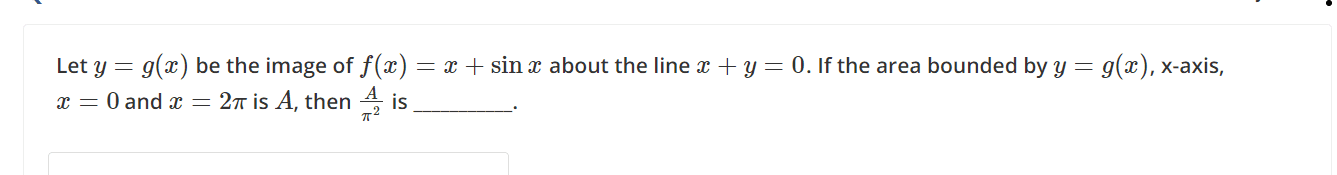# Taking Image of a curve about a given line

• MHB
DaalChawalHow to find image of $f(x)= x + sinx$ about the given line $y = - x$ .

Similarly can we take image of a function about a function? OR is it necessary about which we take image should be a point, line only?

If $y=x+\sin x$, then the reflection around $y=-x$ is $-x=-y+\sin(-y)$ or $x=y+\sin y$. Although a function, this cannot be explicitly solved for $y$. However the two functions are also symmetric around $y=x$ and intersect at the point $(2\pi,2\pi)$. So the area under $g$ between your limits is $2\pi^2$ so your answer is $2$.